Test : Fractions - 1

# Test : Fractions - 1

Test Description

## 10 Questions MCQ Test Mathematics for Class 5: NCERT | Test : Fractions - 1

Test : Fractions - 1 for Class 5 2023 is part of Mathematics for Class 5: NCERT preparation. The Test : Fractions - 1 questions and answers have been prepared according to the Class 5 exam syllabus.The Test : Fractions - 1 MCQs are made for Class 5 2023 Exam. Find important definitions, questions, notes, meanings, examples, exercises, MCQs and online tests for Test : Fractions - 1 below.
Solutions of Test : Fractions - 1 questions in English are available as part of our Mathematics for Class 5: NCERT for Class 5 & Test : Fractions - 1 solutions in Hindi for Mathematics for Class 5: NCERT course. Download more important topics, notes, lectures and mock test series for Class 5 Exam by signing up for free. Attempt Test : Fractions - 1 | 10 questions in 10 minutes | Mock test for Class 5 preparation | Free important questions MCQ to study Mathematics for Class 5: NCERT for Class 5 Exam | Download free PDF with solutions
 1 Crore+ students have signed up on EduRev. Have you?
Test : Fractions - 1 - Question 1

### Represent the shaded part as a fraction and choose the correct option.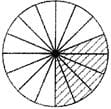Detailed Solution for Test : Fractions - 1 - Question 1

5 parts are shaded in the piechart out of 16
That means fraction of shaded part is 5/16
It represents a proper fraction because it is less than 1
or numerator is less than denominator.

Test : Fractions - 1 - Question 2

### Change the fraction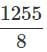into mixed fraction and choose the correct option.

Detailed Solution for Test : Fractions - 1 - Question 2

For option (D) = (156 x 8) + 7 = 1248 + 7 =1255

Test : Fractions - 1 - Question 3

### Which one of the following is not correct?

Detailed Solution for Test : Fractions - 1 - Question 3

A fraction in which the numerator is larger than or equal to the denominator, like 5/2, 17/3, or 6/6 is called an improper fraction.
To put it another way, a fraction with a value less than 1 is a proper fraction.
A mixed Fraction looks like this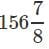and it value is greater than 1.

Test : Fractions - 1 - Question 4

Jack cuts a paper sheet into 56 equal parts, He colours all the parts with different colours. If red parts out of total parts represents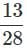, how many parts did jack coloured with red?

Detailed Solution for Test : Fractions - 1 - Question 4

Total no of parts=56
Red parts out of total parts represents = 13/28  That is 13 out of 28
But total cards are 56, So multiply both 13 and 28 by 2, we get
13 x 2 = 26
28 x 2 = 56
Hence from 56 total cards, 26 are coloured red.

Test : Fractions - 1 - Question 5

In which one of the following fractions, unshaded part of the whole figure represents unit fraction?

Detailed Solution for Test : Fractions - 1 - Question 5

D option has only 1 unshaded part out of 5,

Test : Fractions - 1 - Question 6

Which one of the following is an equivalent fraction for 260/312​ ?

Test : Fractions - 1 - Question 7

Which of the following is an example of proper fraction?

Detailed Solution for Test : Fractions - 1 - Question 7

A fraction in which the numerator is smaller than the denominator, like 1/2, 2/3, or 4/6 is called a proper fraction.

Test : Fractions - 1 - Question 8

Convert the mixed fraction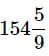​ into improper fraction.

Detailed Solution for Test : Fractions - 1 - Question 8

To convert it multiply 154 x 9 = 1386
Now add 5 to it = 1386 + 5 = 1391 (numerator)
Now the fraction is 1391/ 9

Test : Fractions - 1 - Question 9

Arrange the following fractions in ascending order.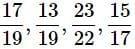Test : Fractions - 1 - Question 10

Which one of the following is not an equivalent fraction of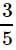?

Detailed Solution for Test : Fractions - 1 - Question 10

A fraction is said to be equivalent fraction only when its simplest form is equal to the actual fraction. For example 12/20 can be considered as an equivalent fraction of 3/5 because:
12/ 20= 3*4/5*4 where 4 /4 =1.So the simplest form is 3/4.
But when we multiply 3*123/5*123
We get answer as 369/ 615
Since the denominator given in question is 625 it is wrong and not an equivalent fraction.

## Mathematics for Class 5: NCERT

36 videos|73 docs|45 tests
 Use Code STAYHOME200 and get INR 200 additional OFF Use Coupon Code
Information about Test : Fractions - 1 Page
In this test you can find the Exam questions for Test : Fractions - 1 solved & explained in the simplest way possible. Besides giving Questions and answers for Test : Fractions - 1, EduRev gives you an ample number of Online tests for practice

## Mathematics for Class 5: NCERT

36 videos|73 docs|45 tests